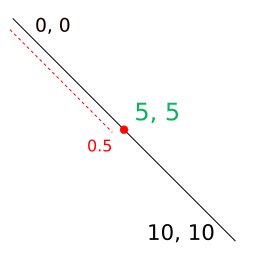# polf’s documentation¶

Simple library written with the Python C API to calculate points on lines. It does not perform any checks on the passed data, but rather follows the GIGO processing pattern.

I have written it with the main purpose of learning, but it may be useful in some situation or it can serve as a reference to get you started in the Python C API.

```pip install polf
```

## Public API¶

polf.line_xy(p0x: float, p0y: float, p1x: float, p1y: float, t: float) list

Computes the coordinate of a point in a 2D rect line parametrized in the range `t` from 0 to 1.

Algorithm: `B(t) = (p0 + p1) * t , 0 <= t <= 1`

Parameters:
• p0x (float) – X value for starting point.

• p0y (float) – Y value for starting point.

• p1x (float) – X value for ending point.

• p1y (float) – Y value for ending point.

• t (float) – Number in the range from 0 to 1 that parametrizes the location in the 2D rect line.

Returns:

Point inside the 2D rect line for the value `t`.

Return type:

list

Example

```>>> line_xy(0, 0, 10, 10, .5)
[5.0, 5.0]
```polf.line_xyz(p0x: float, p0y: float, p0z: float, p1x: float, p1y: float, p1z: float, t: float) list

Computes the coordinate of a point in a 3D rect line parametrized in the range `t` from 0 to 1.

Algorithm: `B(t) = (p0 + p1) * t , 0 <= t <= 1`

Parameters:
• p0x (float) – X value for starting point.

• p0y (float) – Y value for starting point.

• p0z (float) – Z value for starting point.

• p1x (float) – X value for ending point.

• p1y (float) – Y value for ending point.

• p1z (float) – Z value for ending point.

• t (float) – Number in the range from 0 to 1 that parametrizes the location in the 3D rect line.

Returns:

Point inside the 3D rect line for the value `t`.

Return type:

list

Example

```>>> line_xyz(0, 0, 0, 10, 10, 10, .5)
[5.0, 5.0, 5.0]
```polf.cubic_bezier_xy(p0x: float, p0y: float, p1x: float, p1y: float, p2x: float, p2y: float, p3x: float, p3y: float, t: float) list

Computes the coordinate of a point in a 2D cubic Bézier curve parametrized in the range `t` from 0 to 1.

Algorithm: `B(t) = (1-t)**3 * p0 + 3*(1-t)**2 * t * p1 + 3*(1-t)**2 * p2 + t**3 * p3 , 0 <= t <= 1`

Parameters:
• p0x (float) – X value for starting point.

• p0y (float) – Y value for starting point.

• p1x (float) – X value for first control point.

• p1y (float) – Y value for first control point.

• p2x (float) – X value for second control point.

• p2y (float) – Y value for second control point.

• p3x (float) – X value for ending point.

• p3y (float) – Y value for ending point.

• t (float) – Number in the range from 0 to 1 that parametrizes the location in the cubic Bézier curve.

Returns:

Point inside the cubic Bézier curve for the value `t`.

Return type:

list

Example:

```>>> cubic_bezier_xy(0, 10, 0, 0, 10, 0, 10, 10, .5)
[5.0, 2.5]
```polf.quadratic_bezier_xy(p0x: float, p0y: float, p1x: float, p1y: float, p2x: float, p2y: float, t: float) list

Computes the coordinate of a point in a 2D quadratic Bézier curve parametrized in the range `t` from 0 to 1.

Algorithm: `B(t) = (1-t)**2 * p0 + 2*(1-t)*t *p1 + t**2 * p2`

Parameters:
• p0x (float) – X value for starting point.

• p0y (float) – Y value for starting point.

• p1x (float) – X value for control point.

• p1y (float) – Y value for control point.

• p2x (float) – X value for ending point.

• p2y (float) – Y value for ending point.

• t (float) – Number in the range from 0 to 1 that parametrizes the location in the quadratic Bézier curve.

Returns:

Point inside the quadratic Bézier curve for the value `t`.

Return type:

list

polf.elliptical_arc_xy(p0x: float, p0y: float, rx: float, ry: float, x_axis_rotation: float, large_arc: bool, sweep: bool, p1x: float, p1y: float, t: float) list

Computes the coordinate of a point in a 2D elliptical arc parametrized in the range `t` from 0 to 1. This implementation follows SVG2 specification.

Parameters:
• p0x (float) – X value for starting point.

• p0y (float) – Y value for starting point.

• rx (float) – Arc width.

• ry (float) – Arc height.

• x_axis_rotation (float) – Arc rotation on x axis.

• large_arc (bool) – `large-arc` flag that specifies how the arc is drawn.

• sweep (float) – `sweep` flag that specifies how the arc is drawn.

• p1x (float) – X value for ending point.

• p1y (float) – Y value for ending point.

• t (float) – Number in the range from 0 to 1 that parametrizes the location in the elliptical arc.

Returns:

Point inside the elliptical arc for the value `t`.

Return type:

list